Best daily deals

# How does public key cryptography work? - Gary explains

How keys are distributed is vital to any encryption system. Find out how to do it with the Diffie–Hellman key exchange and using public-key cryptography.
By
October 4, 2016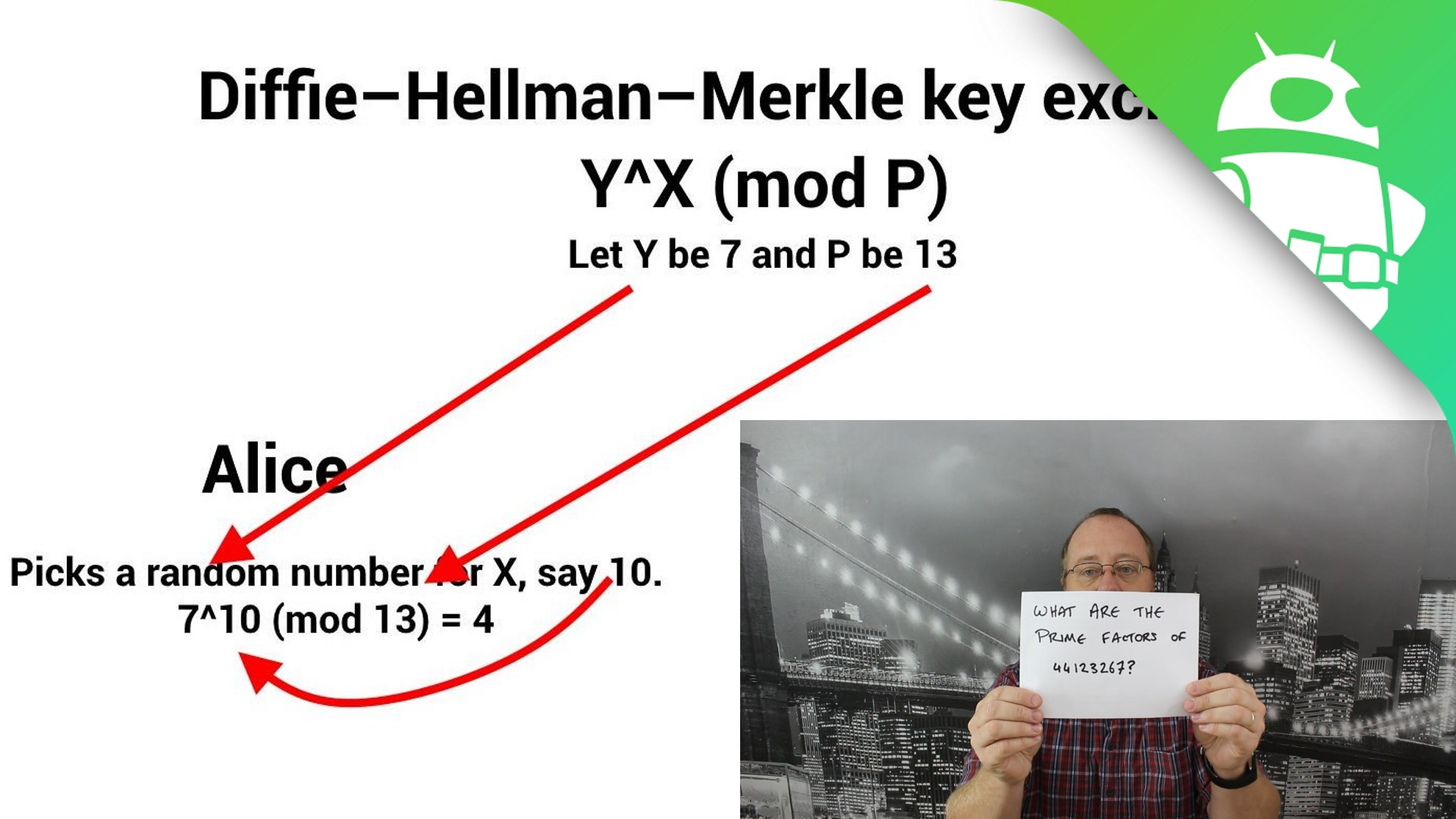In my previous article/video how does encryption work? I wrote about the principles of encryption starting with the Caesar cipher and following the development of cryptography through to the modern day with systems like DES and AES. All these systems of encryption have one thing in common, you need to use a key to encrypt and decrypt the message.

All encryption systems are rendered useless if a third party can discover the key used to encrypt the data. Therefore how keys are passed from one party to another, how keys are distributed is very important. If two people are friends then the issue of key distribution is simple, you meet up in private and swap key information. However if one person is in Europe and the other in North America, how do they exchange the keys without the possibility of a third person eavesdropping? This problem is magnified many times over when we consider the nature of the Internet. All our shopping on Amazon, eBay or wherever is based on the idea that our transactions are protected by encryption. But how does my web browser know what key to use when chatting to Amazon’s servers?

Fortunately the problem of key distribution was cracked nearly 40 years ago in the form of the Diffie–Hellman–Merkle key exchange and then shortly afterwards with the advent of public key cryptography.

## Diffie–Hellman–Merkle key exchange

If Alice and Bob want to communicate securely but they are worried about Eve spying on them, how can Alice and Bob agree on a key for use with a symmetric cipher like DES without Eve finding out the key? That was the question that preoccupied Martin Hellman along with his colleagues Whitfield Diffie and Ralph Merkle during the mid 1970s. After a couple years of head scratching Martin Hellman had a revelation based on the idea of one-way functions. It works like this:

Alice picks a number and so does Bob. Alice picks 10 and Bob picks 2. They have both previously agreed to use the one-way function Y^X  (mod P) where Y is 7 and P is 13, it can be a publicly agreed formula. So Alice plugs her number into the formula and gets: 7^10 (mod 13) = 4. Bob does the same and gets 7^2 (mod 13) = 10.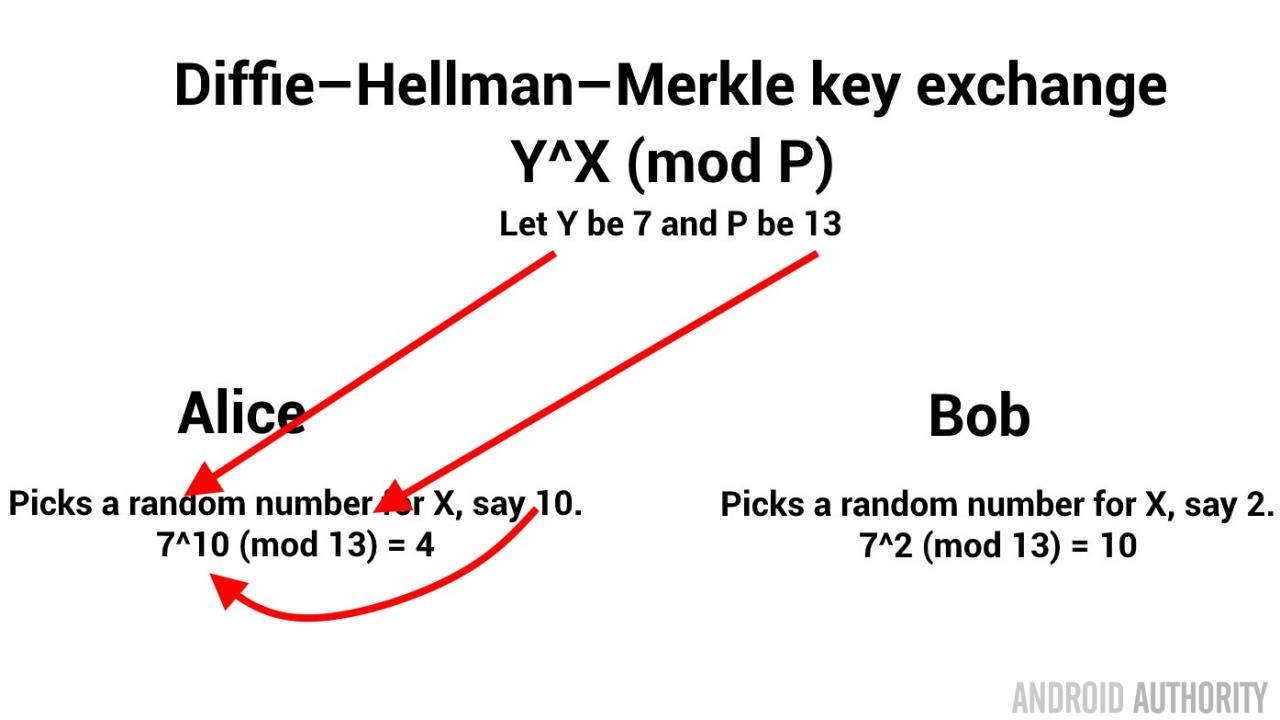At this point Alice send 4 to Bob and Bob sends 10 to Alice. If a third person, Eve, is listening to their exchange then capturing the 4 and the 10 won’t matter, even if she knows the details of the formula 7^X (mod 13). Because trying to guess Alice’s X is hard. There are lots of numbers that result in 4 when plugged into the formula and Eve can’t tell which number it is. For example 7^22 (mod 13) also gives 4. I am using smaller numbers here, but X could be anything.

Now comes the magic. If Alice uses Bob’s 10 as Y and keeps X as 10, the random number she picked, then she gets: 10^10 (mod 13) = 3. Now Bob does the same, Y will be 4 from Alice and X will remain as 2: 4^2 (mod 13) = 3.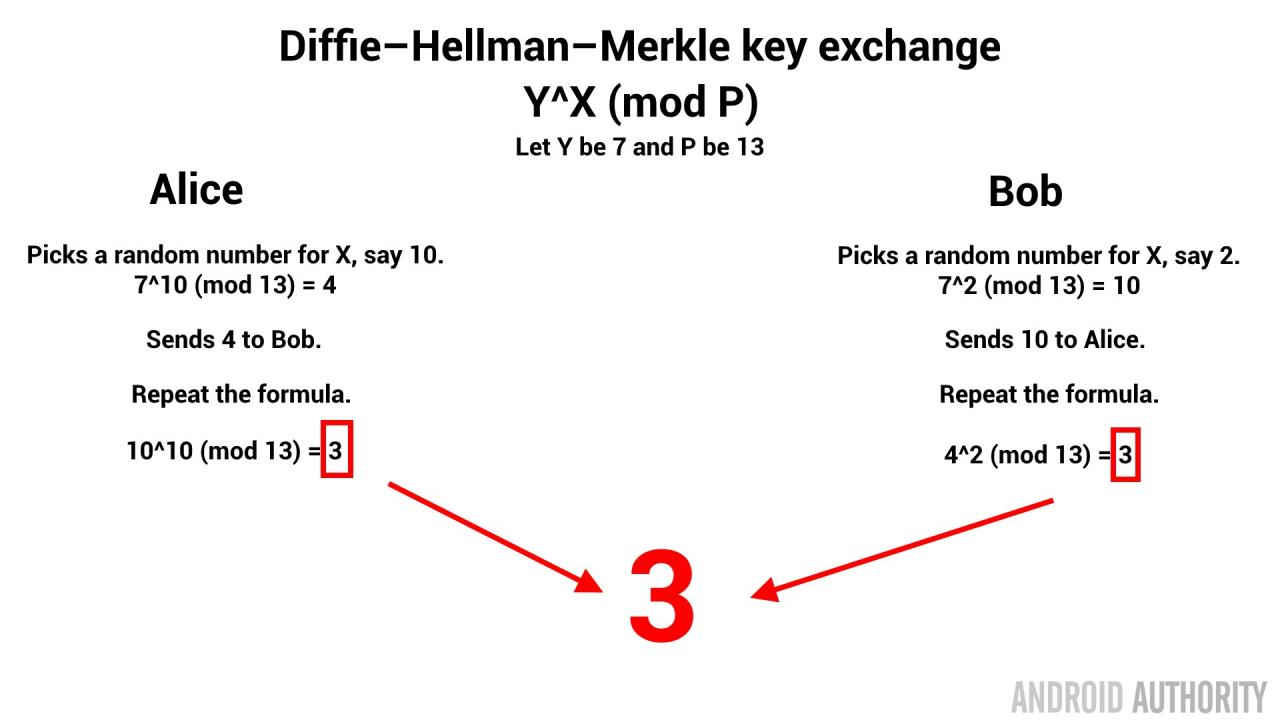JARGON BUSTER
Modular arithmetic (mod or %) – This is a mathematical operation that gives the reminder when two integers are divided. So, 11 divided by 5 = 2 remainder 1. In modular arithmetic that would be 11 mod 5 = 1. Modular arithmetic is great for encryption as it the basis of one-way functions, i.e. functions which are easy to calculate in one direction, but hard (impossible) to reverse.

We know that 11 mod 5 = 1, but so is 22 mod 7, and so is 1729 mod 288. This means that knowing the answer, 1, doesn’t help find the original numbers.

At first it might seem that it isn’t an important idea, however as we can see from the Diffie–Hellman–Merkle key exchange and from RSA, it is in fact a very important notion!

So now both Alice and Bob have the number 3 but Alice never told Bob here random number (10) and Bob never told Alice his random number (2). But yet they both now agree on the key (3) for encryption. Obviously the single digit number 3 is a weak key, however this can be done with large numbers.

Here is an example with larger numbers. Y is 2087 and P is 7703. Alice picks 8001 and Bob picks 12566:

• Alice: 2087^8001 (mod 7703) = 6256
• Bob: 2087^12566 (mod 7703) = 7670

Alice and Bob exchange 6256 and 7670.

• Alice: 7670^8001 (mod 7703) = 3852
• Bob: 6256^12566 (mod 7703) = 3852

Now Bob and Alice agree on the key 3852 and even if Eve can see all the numbers that are exchanged, she can’t guess the key that Bob and Alice are using. For bigger (stronger) keys you just need to use bigger (longer) numbers.

## Asymmetric ciphers

[related_videos title=”Gary also Explains:” align=”left” type=”custom” videos=”718737,714753,699914,699887,694411,681421″]The cryptography that we have discussed until now is known as symmetric, meaning that you use the same key to encrypt some data and then you perform the reverse operation with the same key to decrypt it. There is a symmetry both in the algorithms and the keys. However, there is a different approach. As a result of his work developing a method for secure key exchange, Whitfield Diffe (along with Martin Hellman) developed the idea of asymmetric ciphers. A form of cryptography where one key and algorithm is used to encrypt some data but a different key and algorithm is used to decrypt it. If such an encryption system was possible then it would mean Alice could send Bob a message encrypted using one key and Bob could decrypt it using another. The encryption key could be public, free for everyone to see and use, a public key. But the key to decrypt the data would remain secret, held only by Bob, a private key.

Diffie and Hellman published their ideas in a paper called “New Directions in Cryptography.” The open line of the paper read, “WE STAND TODAY on the brink of a revolution in
cryptography.” And they were right!

While Diffe and Hellman came up with the idea of asymmetric encryption (or public key cryptography), their paper didn’t outline practical way to actually do it. The actual algorithms needed to make public key cryptography possible were discovered by Ronland Rivest while working with Adi Shamir and Leonard Adleman. The discovery led to the development of the popular public-key cryptosystems, RSA (Rivest Shamir Adleman).

The idea is this. If you take two large prime numbers and multiple them together you get the product. It is an easy operation. However to go from the product back to the two prime numbers, when you don’t know either of those numbers, is harder. When I say harder, I don’t mean it is difficult in terms of the maths, that part is easy. If I gave you the number 15 and asked for the prime factors you could quick tell me it was 3 and 5. The maths isn’t hard. However if I have you a very big number, say 44123267, could you tell me prime factors? With a pen and paper it would be hard. With a computer you could write a program that could work it out in a short time. The answer is 7691 x 5737 in case you were interested. Now image we used a number with 300 digits in it. How long would it take a computer to calculate the prime factors?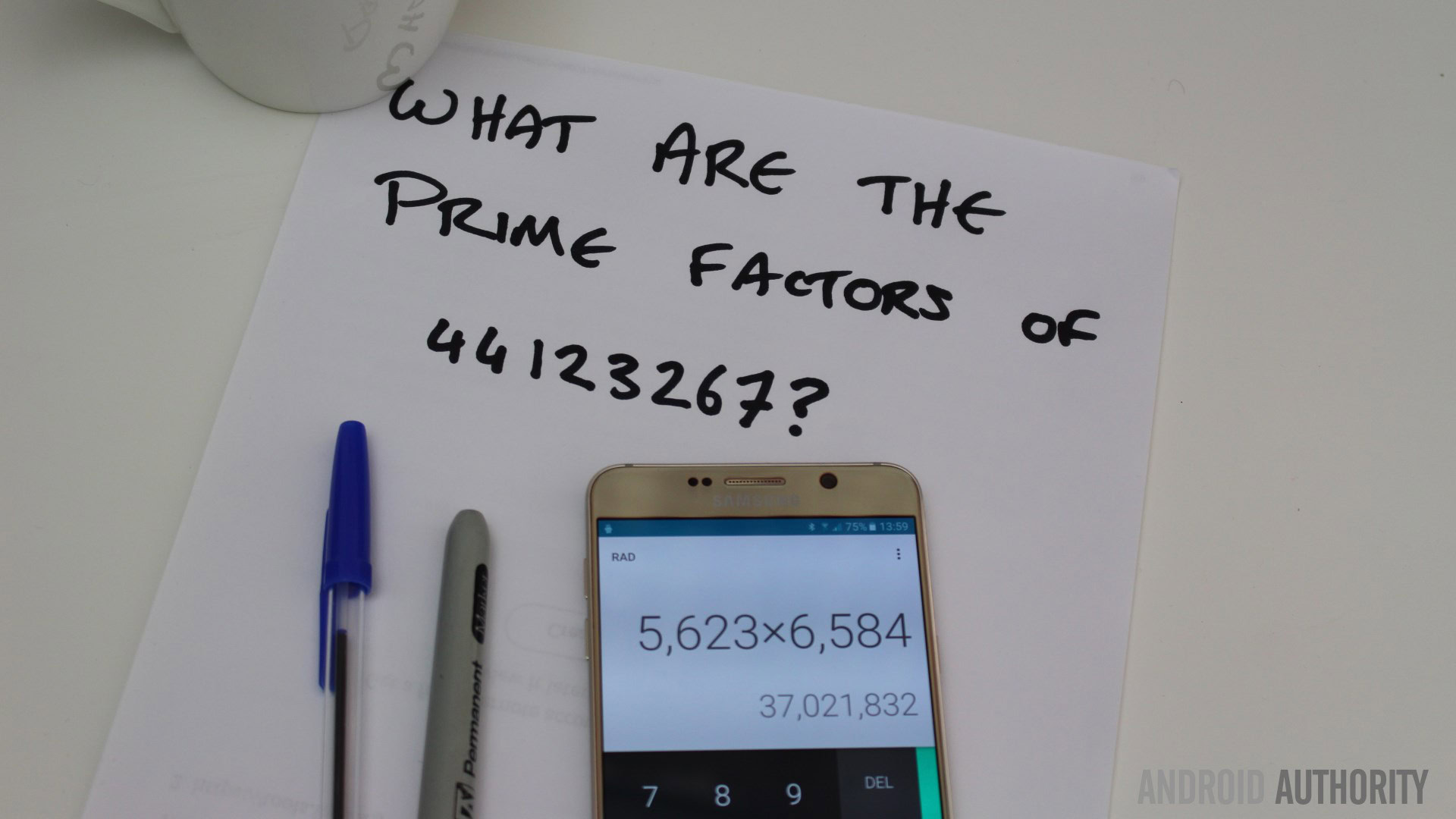The answer is a long time. In 2009, researchers took two years to factor a 232-digit number, using hundreds of computers and the most efficient algorithms. The upshot is that large number factorization is a computationally infeasible. By the way, if you can crack the problem of factorizing and make it as easy as multiplication or addition then you will bring the whole world to its knees!

The difficulty of factoring large numbers means that a message can be encrypted using the product of two large primes (called p and q) as the key in such a way that you need to know p and q to decrypt it. Here is a working of the math for those interested:

• Alice picks two primes p and q. We will use 17 and 19, however in the real world these would be primes with hundreds of digits.
• The product of p and q is 323, this is known as N.
• Another prime, known as e, is chosen. The same value of e is used for everyone, not only Alice and Bob. We will use 7.
• Alice publishes N (and e is already known) so Bob can send her a message.
• If Bob wants to send the message, M, which says “Hello” then “H” has an ASCII value of 72. I will show how to encrypt and decrypt “H”.
• The algorithm to encrypt the text is M^e (mod N). So 72^7 (mod 323) = 13. i.e. 72^7 = 10030613004288. 10030613004288 / 323 = 31054529425 reminder 13.
• Bob sends Alice the number 13.
• If Eve is spying on them and knows N (323) , e (7) and knows the 13 that Bob sent, she can’t work out the value for M. All she knows is that something to the power of 7 (mod 323) has a remainder of 13.
• Alice knows the values of p and q. To decrypt the message she needs to calculate a number called d where (7 * d) (mod ((p-1) * (q-1))) = 1. That is the maths that RSA discovered. So, (7 * d) (mod (16 * 18) = 1. (7 * d) (mod 288) = 1. Deducing d isn’t easy, however with help from Euclid it can be made easier. In this case d is 247. i.e. (7 * 247) (mod 288) = 1.
• To decrypt the message Alice uses, M = C^d (mod N). M = 13^247 (mod 323). M = 72 which is “H” in ASCII.
• Since Eve doesn’t know p or q she can’t perform the same operation, in fact neither can Bob!

## History

It is also worth mentioning that various mathematicians and cryptographers working at the UK Government Communications Headquarters (GCHQ) during the 1970s also developed the idea of “non-secret encryption” (i.e. public key cryptography). The idea was conceived by James H. Ellis in 1970 but he could see no way to implement it. However in 1973, Ellis’ colleague Clifford Cocks implemented what today we call RSA and in 1974, Malcolm J. Williamson developed the same key exchange system as Diffie–Hellman.

Due to the demure nature of GCHQ, and the occasional lack of appreciation for the magnitude of their discoveries, their work was not published at the time. In fact when Diffie and Hellman applied for a patent on their key exchange system the management at GCHQ actively stopped any attempts by Clifford Cocks (and his colleagues) from blocking the patent application by citing prior art.

It wasn’t until 1997 that Clifford Cocks was finally able to divulge his work (and that of Ellis) on key exchange and public-key cryptography.

## HTTPS://

HTTP stands for HyperText Transfer Protocol and with HTTPS the extra “S” on the end means secure, i.e. an encrypted connection. In the past HTTPS used SSL (Secure Sockets Layer) but that has now been replaced by TLS (Transport Layer Security). However since TLS 1.0 used SSL 3.0 as its basis then you often find that the two terms are used interchangeably. What TLS and SSL do is provide the protocol so that encryption can be established between a web browser and a server.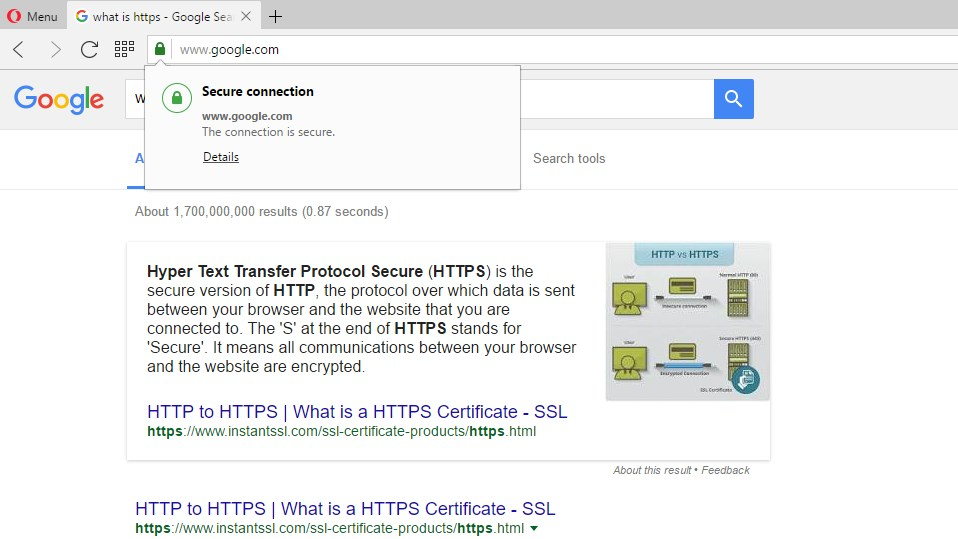When you connect to a remote website that needs a secure connection then your web browser and the server need to agree on a key for the encryption. Using public key cryptography the server is able to advertise its public key (via its digital certificate) and the client can encrypt messages for the server. In fact what happens is that public key cryptography is used to establish a key which is then used for symmetric encryption. However these keys are temporary and last only for one session. TLS also allows for keys to be exchanged using Diffie–Hellman–Merkle.

The important of the digital certificate here is that it verifies that you are connected to the right server and not some rogue server setup to catch you off guard. The certificate contains the public key plus a signature from a signing authority which establishes that this is a valid certificate for the domain.

## Wrap-up

The Diffie–Hellman–Merkle key exchange and public key cryptography (like RSA) have solved the key distribution problem and when used with strong symmetric encryption systems like 3DES or AES then two parties, who have not previously met, can use encryption ensuring that everything from password to payment details remain safe and secure.